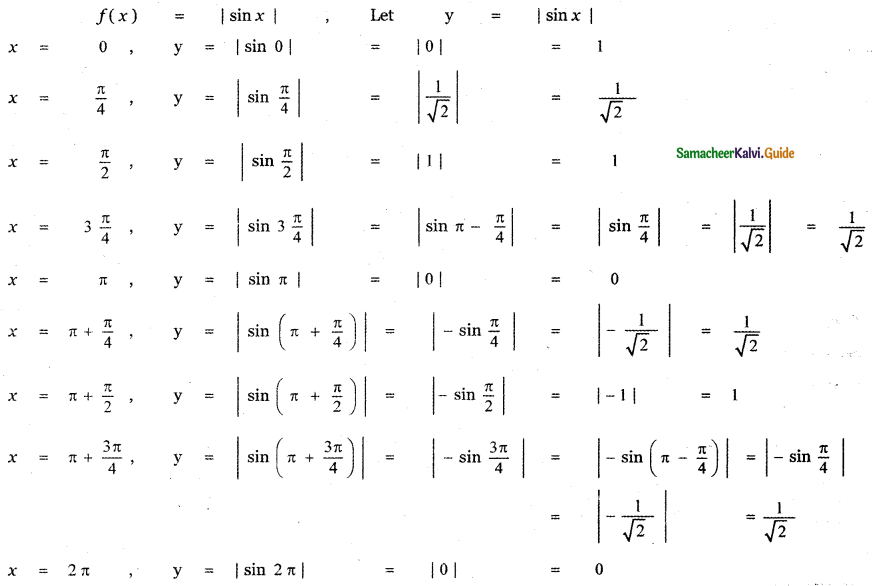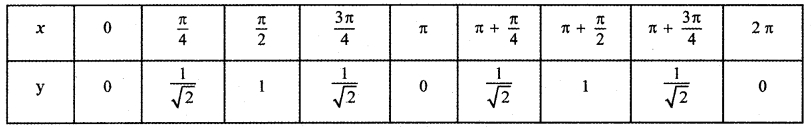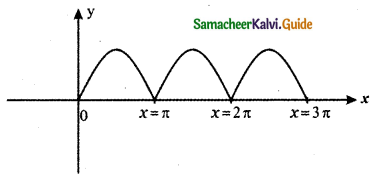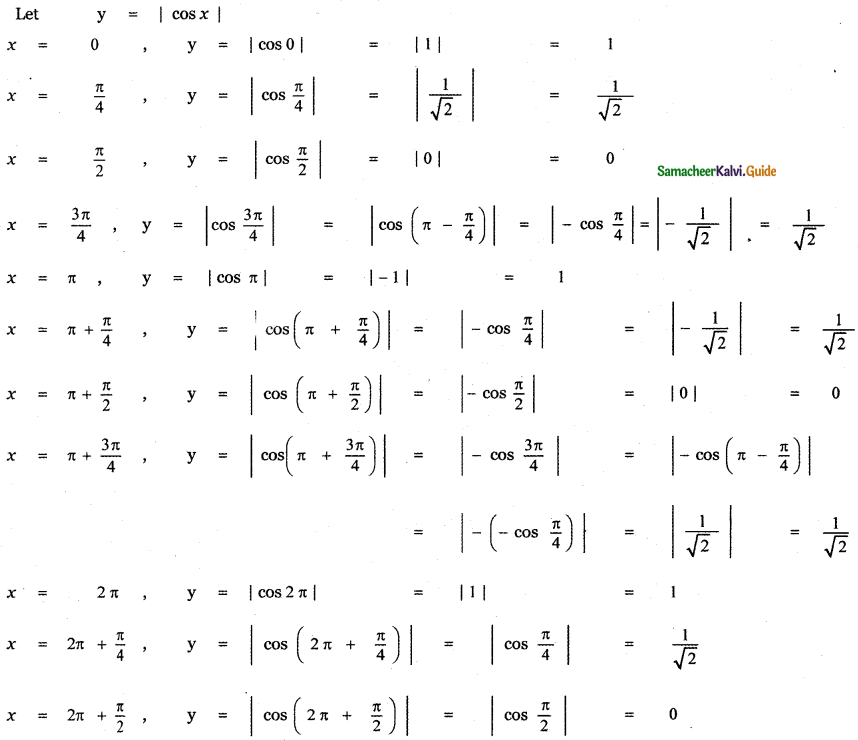Tamilnadu State Board New Syllabus Samacheer Kalvi 11th Maths Guide Pdf Chapter 10 Differentiability and Methods of Differentiation Ex 10.1 Text Book Back Questions and Answers, Notes.

## Tamilnadu Samacheer Kalvi 11th Maths Solutions Chapter 10 Differentiability and Methods of Differentiation Ex 10.1

Question 1.
Find the derivatives of the following functions using the first principle.
(i) f(x) = 6
(ii) f(x) = -4x + 7
(iii) f(x) = -x2 + 2
(i) f(x) = 6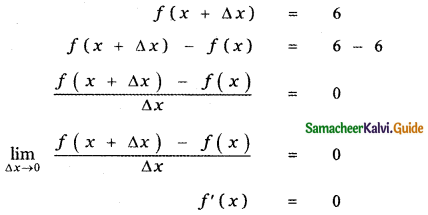(ii) f(x) = – 4x + 7,
f(x + Δx) = -4(x + Δx) + 7
f(x + Δx) – f(x) = [-4(x + Δx) + 7] – [-4x + 7]
f(x + Δx) – f(x) = [-4(x + Δx) + 7] + 4x – 7
f(x + Δx) – f(x) = -4 Δx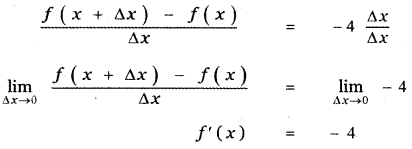(iii) f(x) = -x2 + 2
f (x + Δx) = – (x + Δx)2 + 2
f (x + Δx) – f(x) = – [x2 + 2x Δx + (Δx)2] + 2 – [- x2 + 2]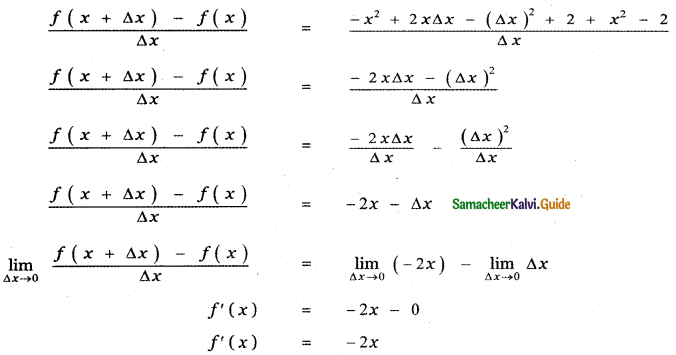Question 2.
Find the derivatives from the left and from the right at x = 1 (if they exist) of the following functions. Are the functions differentiable at x = 1?
(i) f (x) = |x – 1|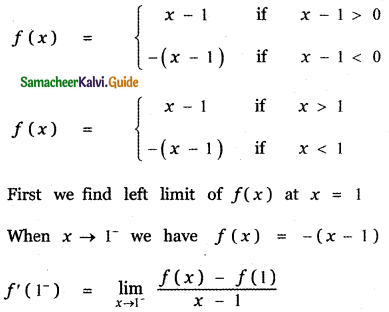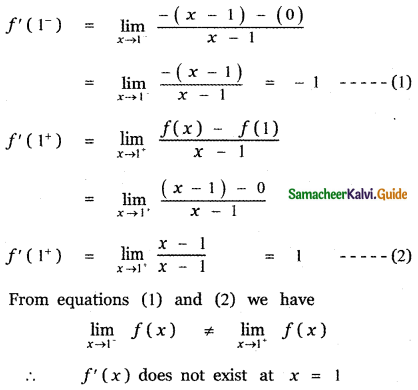(ii) f (x) = $$\sqrt{1-x^{2}}$$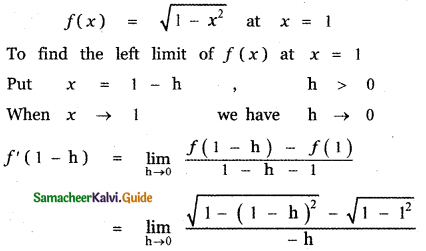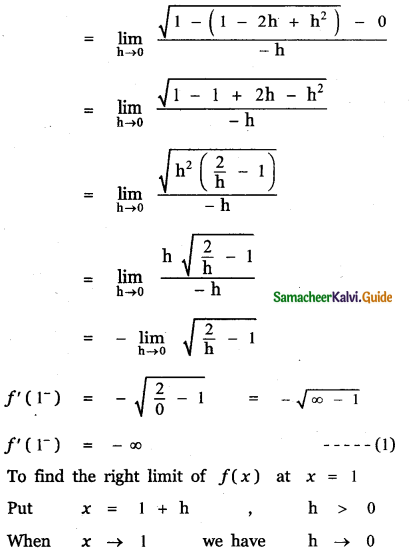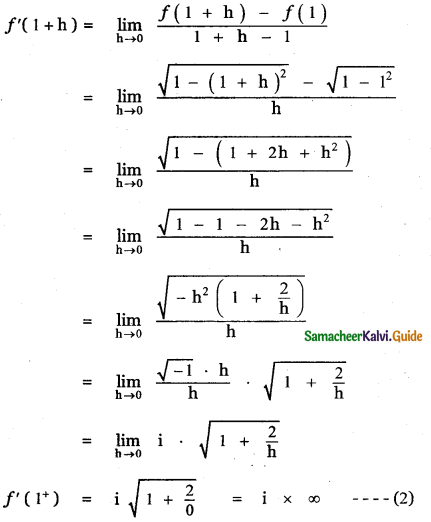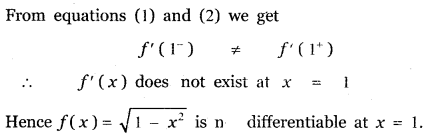(iii)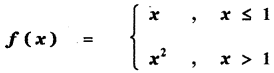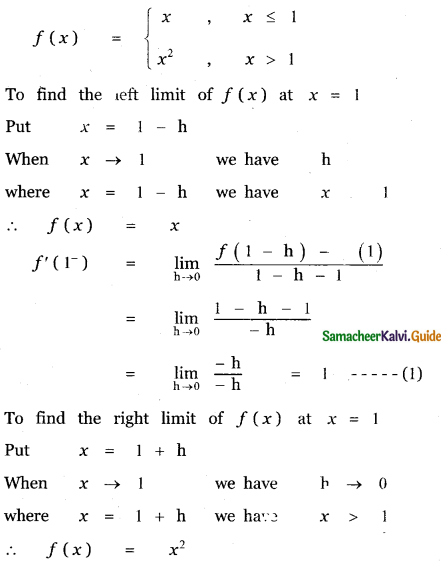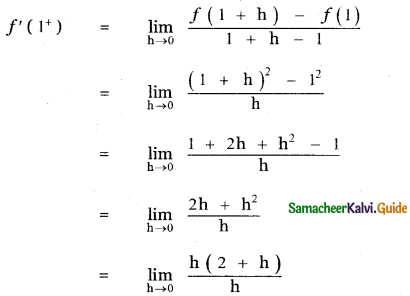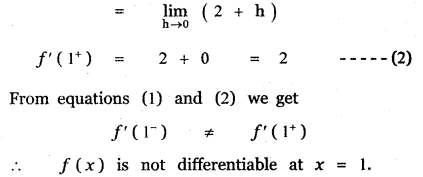Question 3.
Determine whether the following function is differentiable at the indicated values.
(i) f(x) = x |x| at x = 0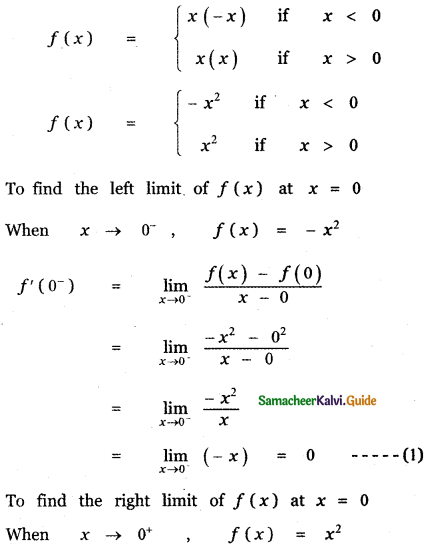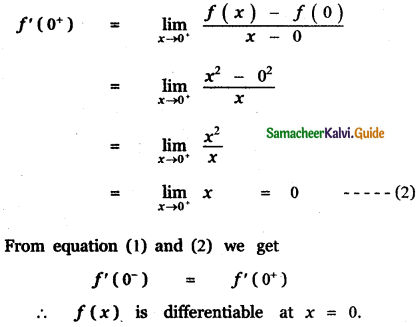(ii) f(x) = |x2 – 1|at x = 1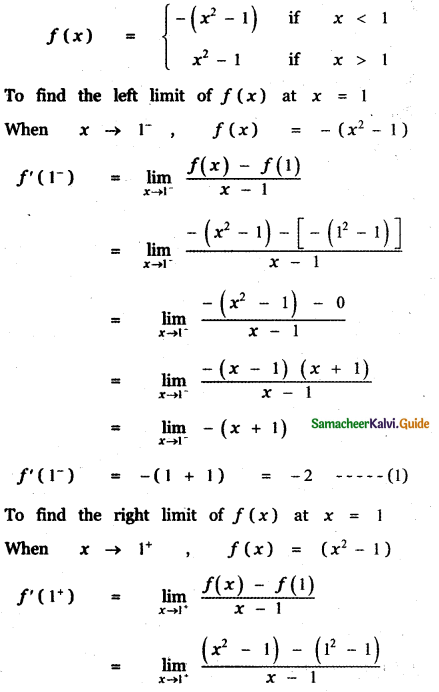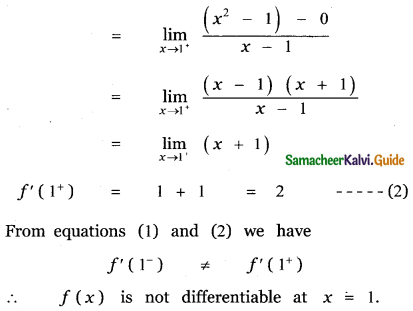(iii) f(x) = |x| + |x – 1| at x = 0, 1
To find the limit at x = 0
First we find the left limit of f(x) at x = 0
When x = 0  |x| = -x and
|x – 1| = -(x – 1)
∴ When x = 0 we have
f(x) = -x – (x – 1)
f(x) = -x – x + 1 = -2x + 1
f(0) = 2 × 0 + 1 = 1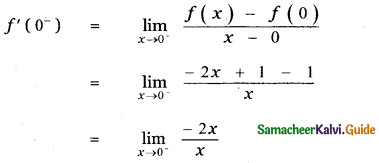f'(0 = – 2 ……… (1)
∴When x = 0+ we have
|x| = x and |x – 1| = – (x – 1)
∴ f(x) = x – (x – 1)
f(x) = x – x + 1
f(x) = 1
f(0) = 1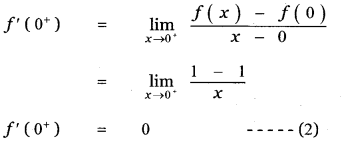From equations (1) and (2) , we get
f'(0) ≠ f’(0+)
∴ f(x) is not differentiable at x = 0.
To find the limit at x = 1
First we find the left limit of f (x) at x = 1
When x = 1 , |x| = x and
|x – 1| = -(x – 1)
∴ f(x) = x – (x – 1)
f(x) = x – x + 1 = 1
f(x) = 1
f(1) = 1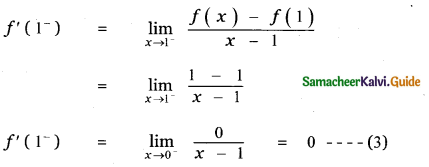When x = 1+ , |x| = x and
|x – 1| = x – 1
When x = , |x| = x and
|x – 1| = x – 1
∴ f(x) = x + x – 1 = 2x – 1
f(1) = 2 × 1 – 1 = 2 – 1 = 1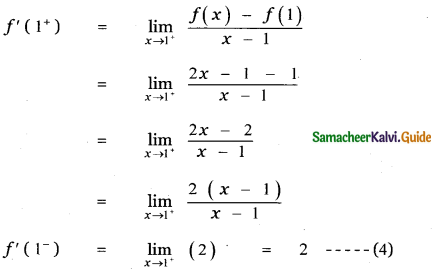From equations (3) and (4) , we get
f’(1) ≠ f'(1+)
∴ f (x) is not differentiable at x = 1(iv) f(x) = sin |x| at x = 0
First we find the left limit of f (x) at x = 0
When x = 0, |x| = -x
∴ f(x) = sin (-x) = -sin x
f(0) = -sin 0 = 0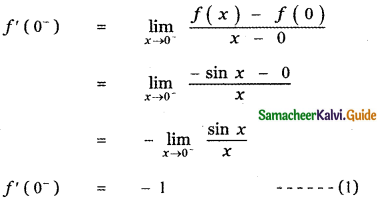Next we find the right limit of f (x) at x = 0
When x = 0+ |x| = x
∴ f(x) = sin x
f(0) = sin 0 = 0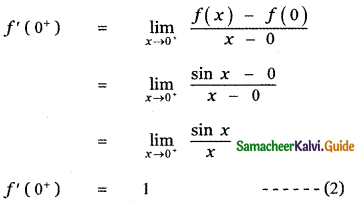From equations (1) and (2) , we get
f’(0) ≠ f'(0+)
∴ f (x) is not differentiable at x = 0.Question 4.
Show that the following functions are not differentiable at the indicated value of x
(i)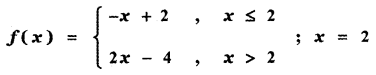First we find the left limit of f(x) at x = 2
When x = 2, then x ≤ 2
∴ f(x) = -x + 2
f(2) = -2 + 2 = 0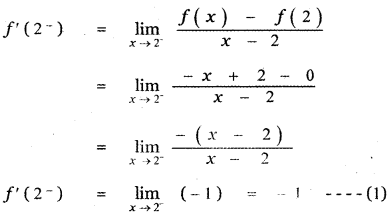Next we find the right limit of f(x) at x = 2
When x = 2+, then x > 2
∴ f(x) = 2x – 4
f(2) = 2 × 2 – 4 = 4 – 4 = 0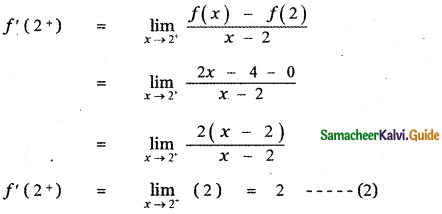From equation (1) and (2), we get
f’(2) ≠ f'(2+)
∴ f (x) is not differentiable at x = 2

(ii)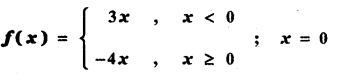First we find the left limit of f (x) at x = 0
When x = 0, then x < 0
∴ f(x) = 3x
f(0) = 3 × 0 = 0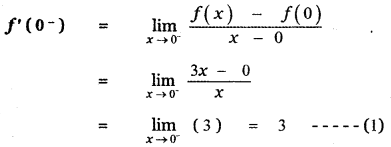Next we find the right limit of f (x) at x = 0
When x = 0+, then x ≥ 0
∴ f(x) = -4x
f(0) = -4 × 0 = 0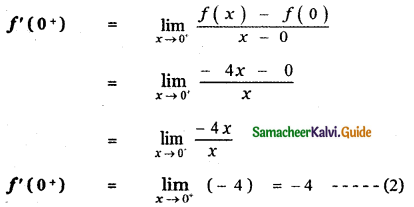From equations (1) and (2) , we get
f'(0) ≠ f'((0+)
∴ f (x) is not differentiable at x = 0Question 5.
The graph of f is shown below. State with reasons that x values (the numbers), at which f is not differentiable.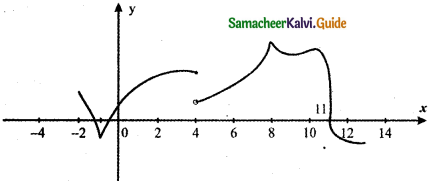We know A function f is not differentiable at a point x0 belonging to the domain of f if one of the following situations holds
(i) f has a vertical tangent at x0
(ii) The graph of f comes to a point at x0 (either a sharp edge ∨ or a sharp peak ∧)
For the given graph f
At x = – 1 , a sharp edge ∨
At x = 8, a sharp peak ∧
At x = 4 , discontinuity
At x = 11, perpendicular tangent
∴ The given graph is not differentiable at
x = – 1, 8, 4, 11

Question 6.
If f(x) = |x + 100| + x2, test whether f’(-100) exists.
f(x) = |x + 100| + x2
First let us find the left limit of f( x) at x = – 1100
When x < – 100 ,
f(x) = – (x + 100) + x2
f(- 100) = – (- 100 + 100) + (- 100)2
f(- 100) = 1002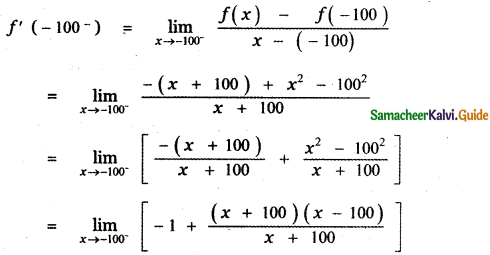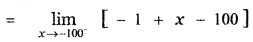= -1 – 100 – 100
f’ (-100) = -201 ——– (1)
Next let us find the right limit of f( x) at x = -100
when x > – 100
f(x) = x + 100 + x2
f(- 100) = – 100 + 100 + (- 100)2
f(- 100) = 1002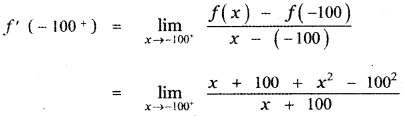f'(-100+) = – 199 ……… (2)
From equation (1) and (2) , we get
f’(- 100) ≠ f'(- 100+)
∴ f’ (x) does not exist at x = -100
Hence, f'(- 100) does not existQuestion 7.
Examine the differentiability of functions in R by drawing the diagrams. (i) |sin x| (ii) |cos x|
(i) |sin x|